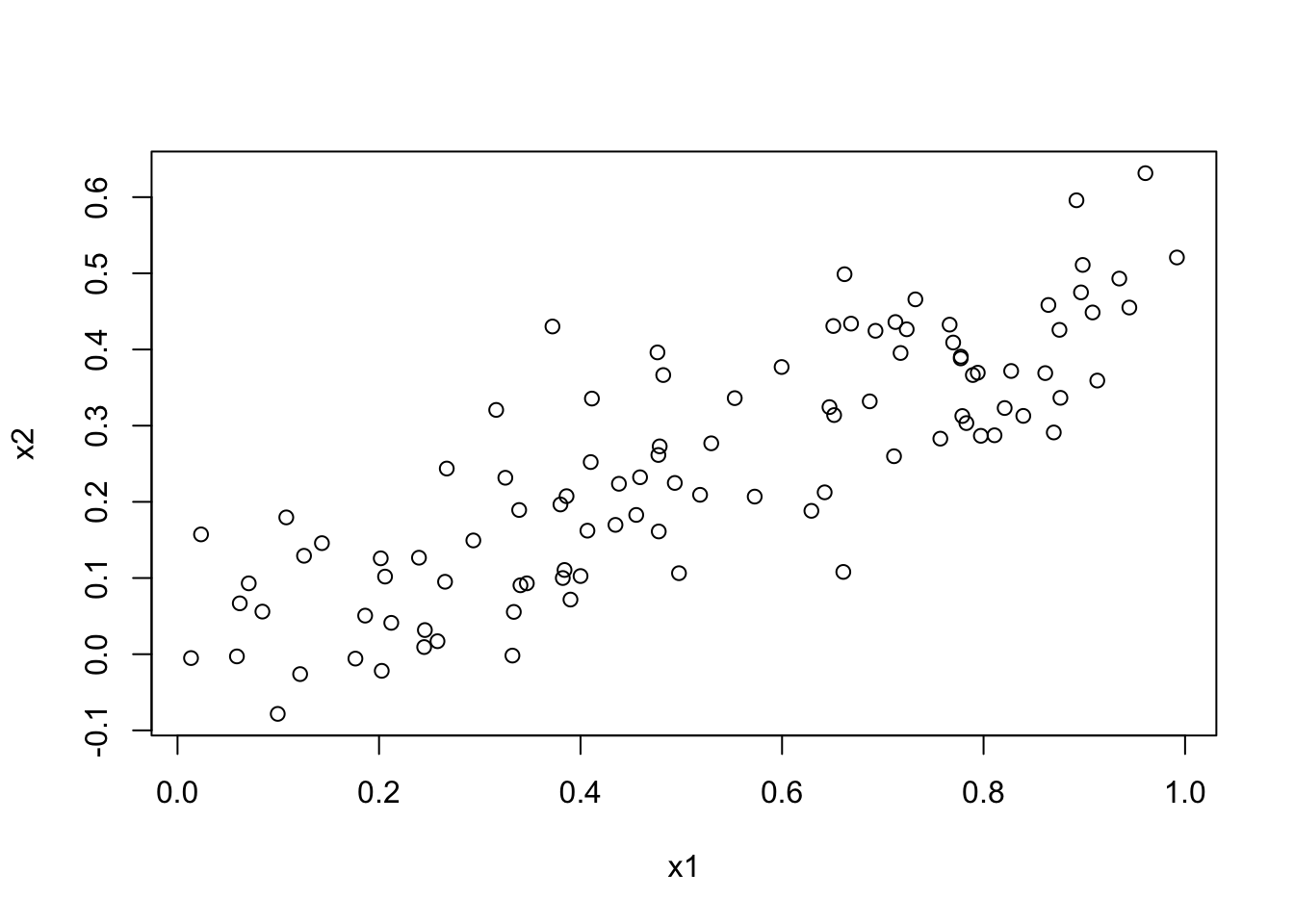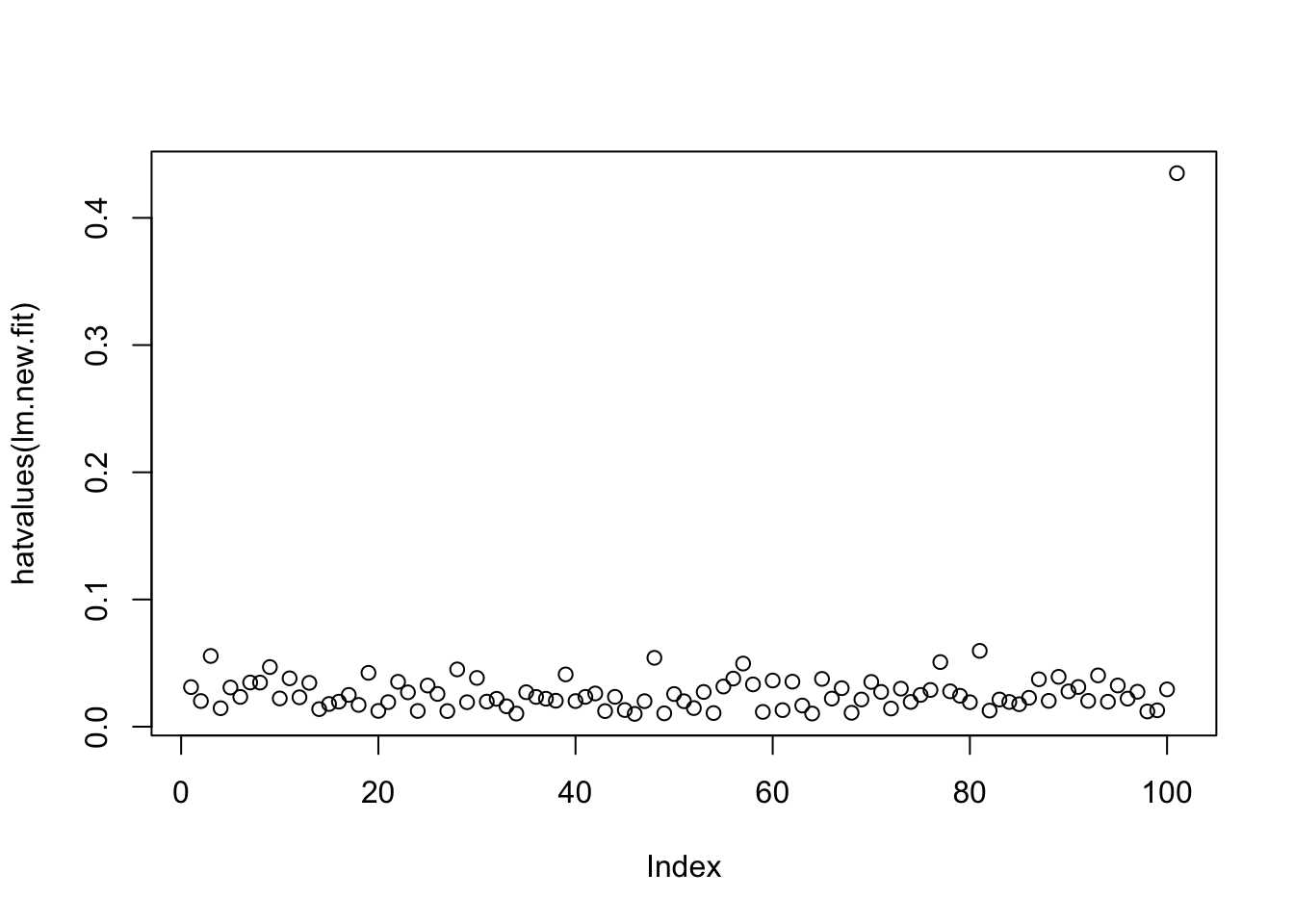ISLR Home

# Question

This problem focuses on the collinearity problem

1. Perform the following commands in R:
> set.seed(1)
> x1=runif(100)
> x2=0.5*x1+rnorm(100)/10
> y=2+2*x1+0.3*x2+rnorm(100)

The last line corresponds to creating a linear model in which y is a function of x1 and x2. Write out the form of the linear model. What are the regression coefficients?

1. What is the correlation between x1 and x2? Create a scatterplot displaying the relationship between the variables.

2. Using this data, fit a least squares regression to predict y using x1 and x2. Describe the results obtained. What are $$\hat{\beta_0}, \hat{\beta_1}$$, and $$\hat{\beta_2}$$? How do these relate to the true $$\beta_0, \beta_1$$, and $$\beta_2$$? Can you reject the null hypothesis $$H_0: \beta_1 = 0$$? How about the null hypothesis $$H_0 : \beta_2 = 0$$?

3. Now fit a least squares regression to predict y using only x1. Comment on your results. Can you reject the null hypothesis $$H_0: \beta_1 = 0$$?

4. Now fit a least squares regression to predict y using only x2. Comment on your results. Can you reject the null hypothesis $$H_0: \beta_1 = 0$$?

6. Now suppose we obtain one additional observation, which was unfortunately mismeasured.

> x1=c(x1, 0.1)
> x2=c(x2, 0.8)
> y=c(y,6)

Re-fit the linear models from (c) to (e) using this new data. What effect does this new observation have on the each of the models? In each model, is this observation an outlier? A high-leverage point? Both? Explain your answers.

# 14a

set.seed(0) # Setting the random seed

Generating x1 data using runif (provides uniform distribution from 0-1)

x1 = runif(100)

Generating data for x2 using random values from normal distribution

x2 = 0.5*x1 + rnorm(100)/10

Creating a linear model. y is a function of x1 and x2

y = 2 + 2*x1 + 0.3*x2 + rnorm(100)
• $$\beta_0 = -2$$
• $$\beta_1 = 2$$
• $$\beta_2 = 0.3$$

# 14b Plotting x1 vs x2

plot(x1, x2)Based on the graph, there is a postive correlation between x1 and x2

## Correlation between x1 and x2

cor(x1, x2) # 0.8324
##  0.8323673

# 14c Fit Model

Fitting the a Least Squares Regression to predict y given x1 and x2

lm.fit = lm(y~x1+x2)

## Model Summary

summary(lm.fit)
##
## Call:
## lm(formula = y ~ x1 + x2)
##
## Residuals:
##     Min      1Q  Median      3Q     Max
## -3.0787 -0.7651 -0.1705  0.8081  2.4605
##
## Coefficients:
##             Estimate Std. Error t value Pr(>|t|)
## (Intercept)   2.0966     0.2289   9.160 8.75e-15 ***
## x1            2.5370     0.7044   3.602 0.000501 ***
## x2           -0.8687     1.1899  -0.730 0.467116
## ---
## Signif. codes:  0 '***' 0.001 '**' 0.01 '*' 0.05 '.' 0.1 ' ' 1
##
## Residual standard error: 1.049 on 97 degrees of freedom
## Multiple R-squared:  0.2345, Adjusted R-squared:  0.2187
## F-statistic: 14.86 on 2 and 97 DF,  p-value: 2.353e-06
• $$\hat{\beta_0} = 2.0966$$
• $$\hat{\beta_1} = 2.5370$$
• $$\hat{\beta_2} = -0.8687$$

The coefficient estimates for $$\hat{\beta_0}$$ and $$\hat{\beta_1}$$ are similar to true coefficients ($$\beta_0$$ and $$\beta_1$$). It is off by at most 0.5 units.

However the coefficient estimate for Bhat2 is off by 1.1 units. Its extremely high p-value (0.4671) tells us that we cannot reject the null hypothesis, which means that there is a high chance that there is no relationship between y and x2.

## VIF to check for multicollinearity

library(car)
## Loading required package: carData
vif(lm.fit)
##       x1       x2
## 3.255583 3.255583

# 14d Creating a new model predicting y using only x1

lm.x1 = lm(y~x1)

## Model Summary

summary(lm.x1)
##
## Call:
## lm(formula = y ~ x1)
##
## Residuals:
##     Min      1Q  Median      3Q     Max
## -3.0009 -0.6889 -0.1621  0.7807  2.5362
##
## Coefficients:
##             Estimate Std. Error t value Pr(>|t|)
## (Intercept)   2.1015     0.2282   9.207 6.36e-15 ***
## x1            2.1089     0.3895   5.415 4.37e-07 ***
## ---
## Signif. codes:  0 '***' 0.001 '**' 0.01 '*' 0.05 '.' 0.1 ' ' 1
##
## Residual standard error: 1.047 on 98 degrees of freedom
## Multiple R-squared:  0.2303, Adjusted R-squared:  0.2224
## F-statistic: 29.32 on 1 and 98 DF,  p-value: 4.373e-07

We can reject the null hypothesis because the p-value (4.37E-7) is extremely below 0.05.

# 14e Creating a new model predicting y using only x2

lm.x2 = lm(y~x2)

## Model Summary

summary(lm.x2)
##
## Call:
## lm(formula = y ~ x2)
##
## Residuals:
##     Min      1Q  Median      3Q     Max
## -2.4823 -0.8368 -0.1330  0.8120  2.5029
##
## Coefficients:
##             Estimate Std. Error t value Pr(>|t|)
## (Intercept)   2.5227     0.2076  12.154  < 2e-16 ***
## x2            2.6985     0.6986   3.863 0.000201 ***
## ---
## Signif. codes:  0 '***' 0.001 '**' 0.01 '*' 0.05 '.' 0.1 ' ' 1
##
## Residual standard error: 1.112 on 98 degrees of freedom
## Multiple R-squared:  0.1321, Adjusted R-squared:  0.1233
## F-statistic: 14.92 on 1 and 98 DF,  p-value: 0.0002014

We can reject the null hypothesis because the p-value (0.0002) is extremely below 0.05.

# 14f

In (c), the p-value for Bhat2 was not siginificant but in (e) if we do a LSR model separately, then it does become significant. Also the coefficient for Bhat2 changed by almost 2 units from (c) and (e).

# 14g

Adding mismeasured observations into the data

x1 = c(x1, 0.1)
x2 = c(x2, 0.8)
y = c(y, 6)

## Refitting the data with new data

lm.new.fit = lm(y~x1+x2)
summary(lm.new.fit)
##
## Call:
## lm(formula = y ~ x1 + x2)
##
## Residuals:
##     Min      1Q  Median      3Q     Max
## -2.8386 -0.7164 -0.2169  0.7466  2.5947
##
## Coefficients:
##             Estimate Std. Error t value Pr(>|t|)
## (Intercept)   2.2092     0.2358   9.368 2.85e-15 ***
## x1            1.2177     0.5870   2.075   0.0407 *
## x2            1.5187     0.9491   1.600   0.1128
## ---
## Signif. codes:  0 '***' 0.001 '**' 0.01 '*' 0.05 '.' 0.1 ' ' 1
##
## Residual standard error: 1.095 on 98 degrees of freedom
## Multiple R-squared:  0.2024, Adjusted R-squared:  0.1862
## F-statistic: 12.44 on 2 and 98 DF,  p-value: 1.536e-05

## Creating a new model predicting y using only x1

lm.new.x1 = lm(y~x1)

## Model Summary

summary(lm.new.x1)
##
## Call:
## lm(formula = y ~ x1)
##
## Residuals:
##     Min      1Q  Median      3Q     Max
## -2.9627 -0.7071 -0.1782  0.7696  3.5646
##
## Coefficients:
##             Estimate Std. Error t value Pr(>|t|)
## (Intercept)   2.2453     0.2366   9.491 1.41e-15 ***
## x1            1.9013     0.4056   4.687 8.86e-06 ***
## ---
## Signif. codes:  0 '***' 0.001 '**' 0.01 '*' 0.05 '.' 0.1 ' ' 1
##
## Residual standard error: 1.103 on 99 degrees of freedom
## Multiple R-squared:  0.1816, Adjusted R-squared:  0.1733
## F-statistic: 21.97 on 1 and 99 DF,  p-value: 8.861e-06

## Creating a new model predicting y using only x1

lm.new.x2 = lm(y~x2)

## Model Summary

summary(lm.new.x2)
##
## Call:
## lm(formula = y ~ x2)
##
## Residuals:
##     Min      1Q  Median      3Q     Max
## -2.5156 -0.8391 -0.1422  0.7896  2.5563
##
## Coefficients:
##             Estimate Std. Error t value Pr(>|t|)
## (Intercept)   2.4708     0.2025  12.199  < 2e-16 ***
## x2            2.9518     0.6616   4.462 2.15e-05 ***
## ---
## Signif. codes:  0 '***' 0.001 '**' 0.01 '*' 0.05 '.' 0.1 ' ' 1
##
## Residual standard error: 1.113 on 99 degrees of freedom
## Multiple R-squared:  0.1674, Adjusted R-squared:  0.159
## F-statistic: 19.91 on 1 and 99 DF,  p-value: 2.152e-05

Given the new data, it makes the $$R^2$$ worse and also the coefficient estimate for Bhat1 is farther that before.

## Checking for leverage

plot(hatvalues(lm.new.fit))Based on the graph above, the new data point has high leverage

## Diagnostic Plots

par(mfrow = c(2,2)) # 4 plots in same picture
plot(lm.new.fit)